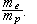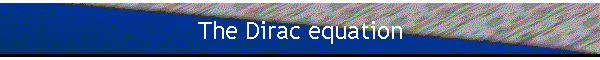The Dirac equation for a spin ½ particle is of the form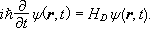For a free particle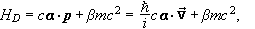with ax2=ay2=az2=b2=1 and all four quantities ax, ay, az, and b anti-commuting in pairs.

For example axay+ayax=0.

Since a and b anti-commute, they cannot be numbers.  For a spin ½ particle ax, ay, az, and b are represented by 4´4 matrices.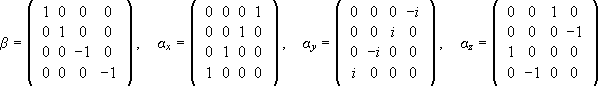In compact notation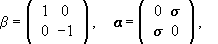where each entry is a 2´2 matrix.

Given a and b as 4´4 matrices, y(r,t) must be a matrix with 4 rows and 1 column.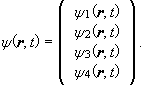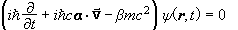is equivalent to 4 coupled first order, linear, homogeneous, partial differential equations for the 4 y’s.  Plane wave solutions of the form yj(r,t)=ujexp(i(k ×r-wt)), j=1,2,3,4 can be found, where the uj are numbers.  These are eigenfunctions of the energy operator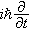and momentum operator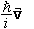with eigenvalues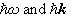respectively.

Substituting these plane wave solutions intoyields a set of algebraic equations for the uj, where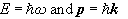are now numbers, not operators..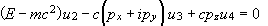.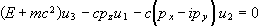..

These equations are homogeneous in the uj and have a solution only if the determinant of the coefficients is zero.  This determinant equals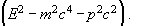Explicit solutions can be obtained for any p by choosing a sign for the energy, say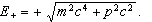Then there are two linearly independent solutions, which are conveniently written as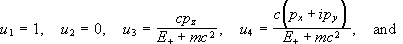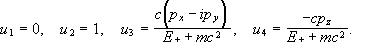If we choose the negative square root,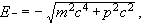then we obtain two different solutions, which are written as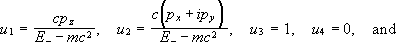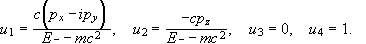Each of these 4 solutions can be normalized in the sense that yy*=1 by dividing it by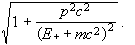In the non relativistic limit E+ is close to mc2 and much larger than c|p| and for the positive energy solutions u3 and u4 are approximately a factor v/c smaller than u1 or u2.

The Dirac Hamiltonian for a spin ½ particle in the presence of an electromagnetic field is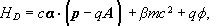and the time independent Dirac equation may be written as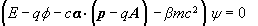in SI units.  For a particle with charge q in a spherically symmetric scalar potential we have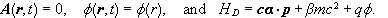#### The Dirac equation for an electron in the field of a proton

Assume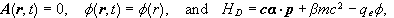i.e. neglect the spin of the proton.  (qe=1.6×10-19C)

Assume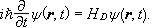Then the orbital angular momentum L is not a constant of motion.

In the Heisenberg picture we have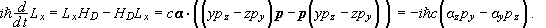Let us define the operator s’, which is represented by the matrixThe operator s’ is not a constant of motion.  We have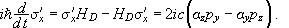But we can see that the operator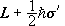is a constant of motion. It commutes with HD.  This operator is interpreted as the total angular momentum operator.  The operator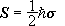is referred to as the spin angular momentum operator.

Let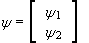in compact notation, both y1 and y2 having two components.  We may then write the eigenvalue equation for HD ,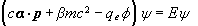as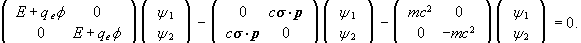This yields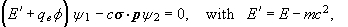and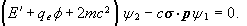We can use the second equation to obtain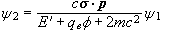and substitute this expression for y2 into the first equation. We the obtain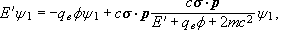or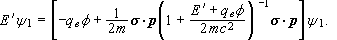In the non relativistic limit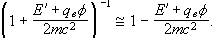In the above equation p is an operator,Therefore pf is an operator, which may be written as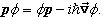We may write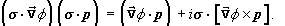using the general result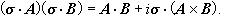[We can apply this equation to the operators since s commutes with p and the gradient of f, and the order of p and the gradient of f is not changed.]

Therefore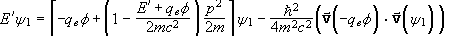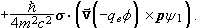For a spherically symmetric potential  V=-qef   we have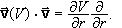In the non relativistic limit we also have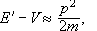and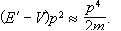Therefore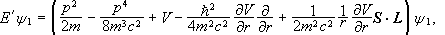where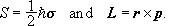The first and third term give the non relativistic Schroedinger equation, the second term has the form of the classical relativistic correction, the last term is the spin-orbit energy, which appears as an automatic consequence of the Dirac equation.  The fourth term is a similar relativistic correction to the potential energy, which does not have a classical analog.  It is called the Darwin term.
The ratio of < all the correction terms > to <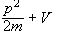> is on the order of v2/c2.

[Note that y1 is the two component spinor as used in the non relativistic description of a spin ½ particle.]

If we do not neglect the spin and the magnetic moment of the proton, we cannot set A(r,t)=0.  We now have to replace p by (p-qA), where q=-qe.  We obtain the equation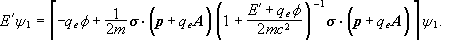We assume that < qeA > << <p> and only keep terms to the lowest order in qeA.  We also neglect terms that containThen only one new term is added to our equation for E’ derived when A(r,t)=0.  We find the new term by evaluating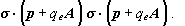We have in generalTherefore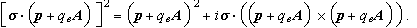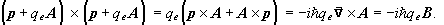[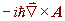is an operator. When it operates on a wavefunction y we have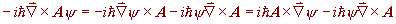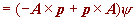]

The new term therefore is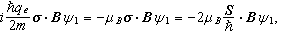andThe first term contains the spin-orbit interaction energy of the proton and the last term the spin-spin interaction energy.  Both terms are hyperfine structure terms.

The hyperfine structure Hamiltonian (neglecting terms in (qA)2) is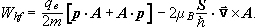It may be rewritten as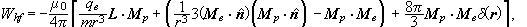since A is the vector potential due to the magnetic moment of the proton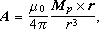and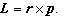(See Cohen-Tannoudji, Complement AXII.)

The fine structure Hamiltonian is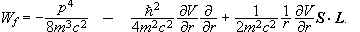H = H0 + Wf + Whf.

The ratio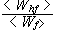is on the order of